# 模型的独立学习方式

### 集成学习

#### Bagging类方法

Bagging类方法是通过随机构造训练样本、随机选择特征等方法来提高每个基模型的独立性，代表性方法有Bagging和随机森林。

• Bagging（Bootstrap Aggregating）是一个通过不同模型的训练数据集的独立性来提高不同模型之间的独立性。我们在原始训练集上进行有放回的随机采样，得到M比较小的训练集并训练M个模型，然后通过投票的方法进行模型集成。
• 随机森林（Random Forest）是在Bagging的基础上再引入了随机特征，进一步提升每个基模型之间的独立性。在随机森林中，每个基模型都是一棵树。

• 从样本集中通过重采样的方式产生n个样本
• 假设样本特征数目为a，对n个样本选择a中的k个特征，用建立决策树的方式获得最佳分割点
• 重复m次，产生m棵决策树
-多数投票机制来进行预测

#### Boosting类方法

Boosting类方法是按照一定的顺序来先后训练不同的基模型，每个模型都针对前续模型的错误进行专门训练。根据前序模型的结果，来提高训练样本的权重，从而增加不同基模型之间的差异性。Boosting类方法的代表性方法有AbaBoost，GBDT，XGB，LightGBM等。

$F(x) = \sum_{m=1}^{M} a_m f_m(x)$

Boosting类方法的关键是如何训练每个弱分类器$f_m(x)$以及对应的权重$a_m$。为了提高集成的效果，应尽可能使得每个弱分类器的差异尽可能大。一种有效的方法是采用迭代的策略来学习每个弱分类器，即按照一定的顺序依次训练每个弱分类器。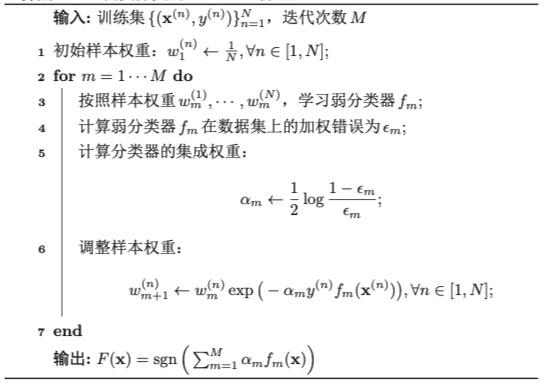$L(F) = exp(-y F(x)) \\ = exp(-y \sum_{m=1}^{M} a_m f_m(x))$

$F_{m-1}(x) = \sum_{t=1}^{T} a_t f_t(x)$

$L(a_m,f_m(x)) = \sum_{n=1}^{N}exp(-y^{(n)} (F_{m-1} (x^{(n)}) + a_m f_m(x^{(n)})))$
$w_m^{(n)}=exp(-y^{(n)} F_{m-1}(x^{(n)}))$，则损失函数可以表示为：
$L(a_m,f_m(x)) = \sum_{n=1}^{N} w_m^{(n)} exp(-y^{(n)} a_m f_m(x^{(n)}))$

$yf_m(x) = 1-2I(y\neq f_m(x))$

$L(a_m, f_m(x)) = \sum_{n=1}^{N} w_m^{(n)} ( 1 - a_m y^{(n)}f_m(x^{(n)}) + \frac{1}{2}a_m^2 ) \\ \propto a_m \sum_{n=1}^{N} w_n^{(n)} I(y^{(n} \neq f_m(x^{(n)})$

$L(a_m, f_m(x)) = \sum_{y^{(n)}=f_m(x^{(n)})} w_m^{(n)} exp(-a_m) + \sum_{y^{(n)} \neq f_m(x^{(n)})} w_m^{(n)} exp(a_m) \\ \propto (1-\epsilon _m) exp(-a_m) + \epsilon_m exp(a_m)$

$\epsilon_m = \frac { \sum_{ y^{(n)} \neq f_m(x^{(n)}) } w_m^{(n)} } {\sum_{n} w_m^{(n)}}$

$a_m = \frac {1}{2} log \frac {1-\epsilon_m}{\epsilon_m}$

• 作为分类器精度很高
• 使用简单分类器时，计算出的结果可理解，且构造简单
• 不需要做特征筛选
• 不同担心过拟合

• 容易收到噪声干扰
• 训练时间长，因为需要遍历所有特征
• 执行效果依赖于弱分类器的选择

### 自训练和协同训练

#### 自训练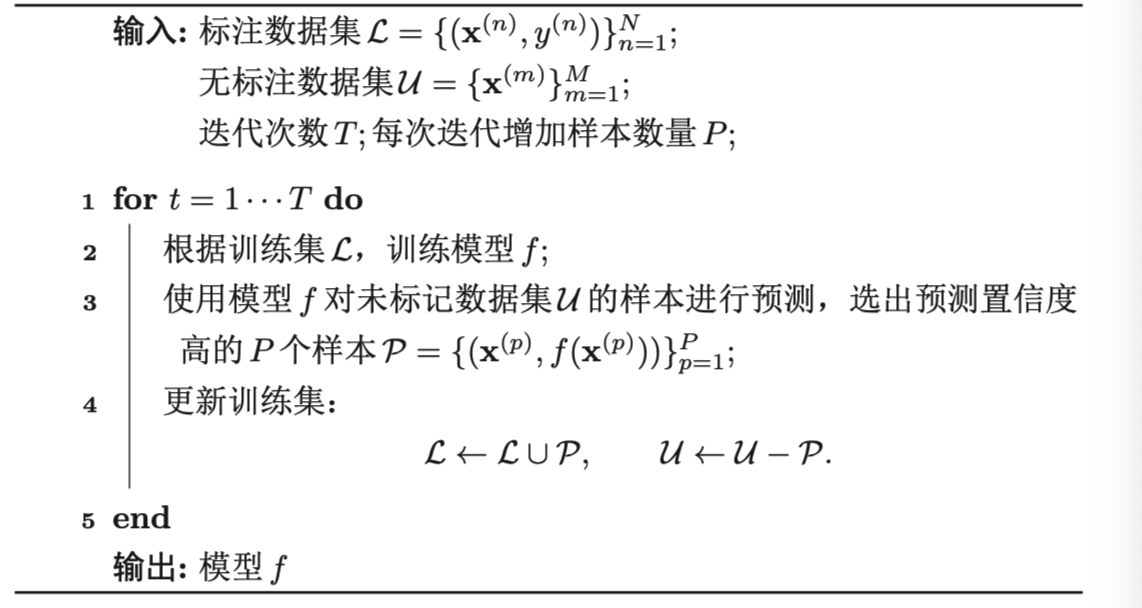#### 协同训练

• （1）：条件独立性，即给定样本标签y时，两种特征条件独立$p(x_1,x_2|y)=p(x_1|y)p(x_2|y)$
• （2）：充足和冗余性，即当数据充分时，每种视角的特征都可以足以单独训练出一个正确的分类器。

$y=g(x)$为需要学习的真实映射函数，$f_1$$f_2$分别为两个视角的分类器，有：
$\exists f_1, f_2, \forall x \in X,\,\,\,\,\, f_1(x_1) = f_2(x_2) = g(x)$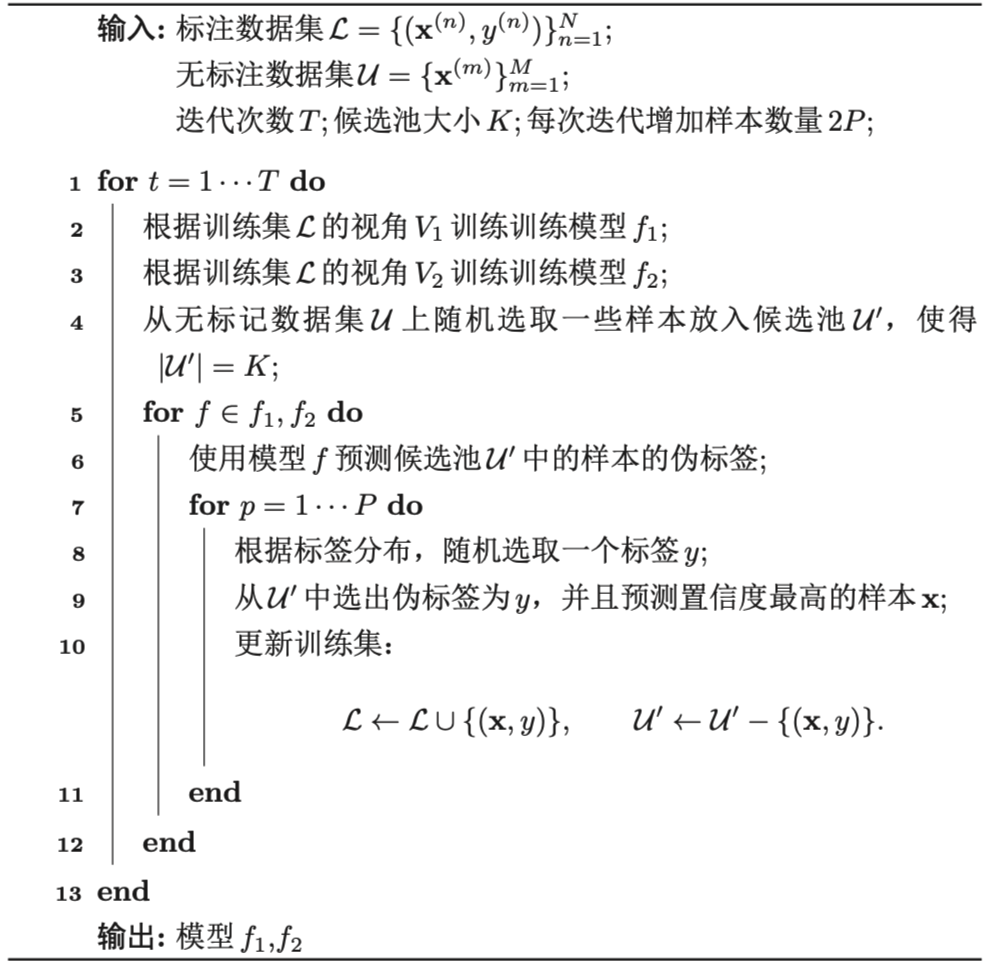### 多任务学习

• 硬共享模式：让不同任务的神经网络模型共同使用一些共享模块来提取一些通用的特征，然后再针对每个不同的任务设置一些私有模块来提取一些任务特定的特征。
• 软共享模式：不显式设置共享模块，但每个任务都可以从其他任务中“窃取”一些信息来提高自己的能力。窃取的方式包括直接复制使用其他任务的隐状态，或使用注意力机制来主动选择有用的信息。
• 层次共享模式：一般神经网络中不同层抽取的特征类型不同，底层一般抽取一些低级的局部特征，高层抽取一些高级的抽象语义特征。因此如果多任务学习中不同任务也有级别高低之分，那么一个合理的共享模式是让低级任务在底层输出，高级任务在高层输出。
• 共享-私有模式：一个更加分工明确的方式是将共享模块和任务特定（私有）模块的责任分开。共享模块捕捉一些跨任务的共享特征，而私有模块只捕捉和特点任务相关的特征。最终的表示由共享特征和私有特征共同构成。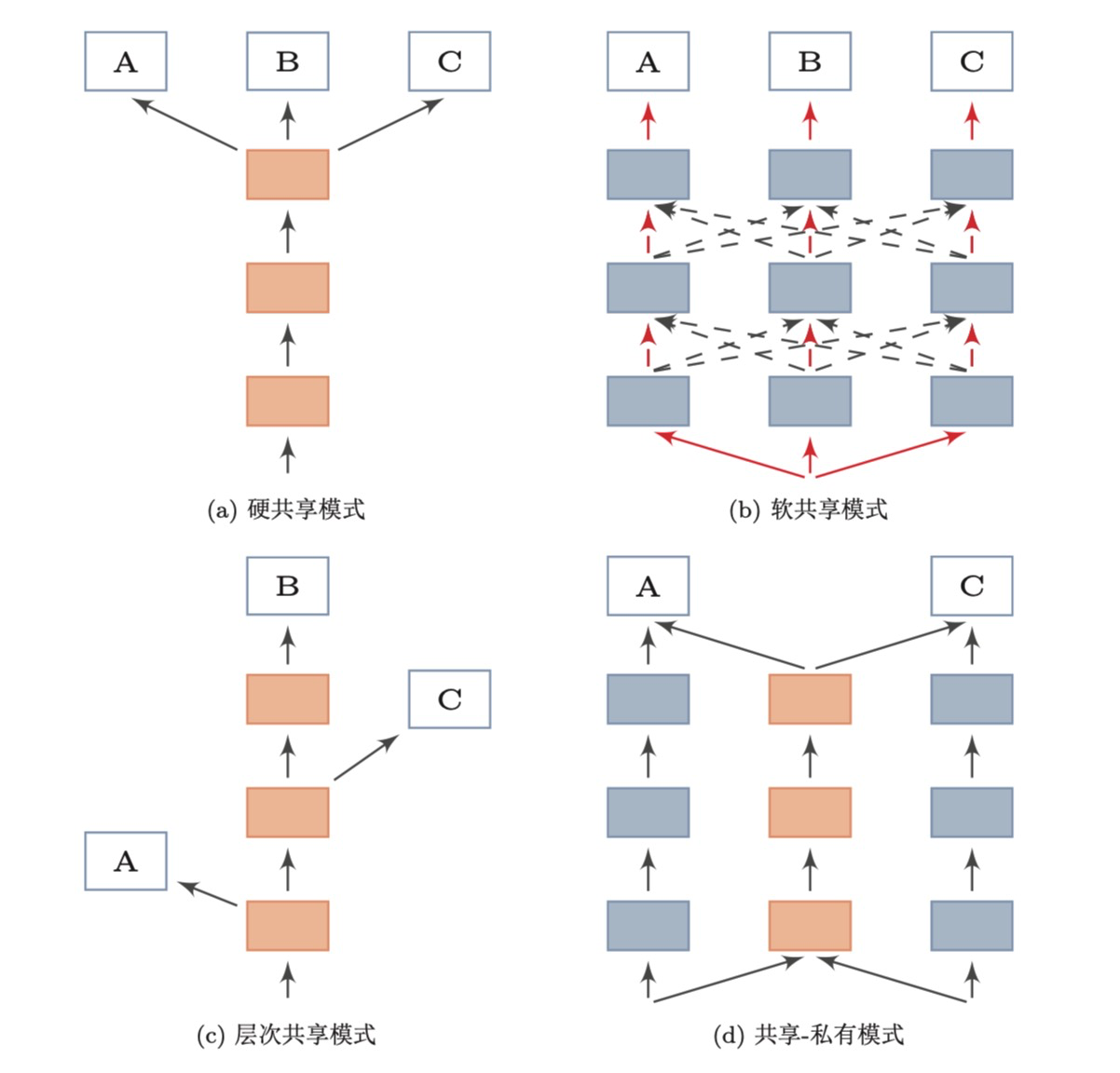• （1）联合训练阶段：每次迭代时，随机挑选一个任务，然后从这个任务中随机选择一些训练样本，计算梯度并更新参数
• （2）单任务精调阶段：基于多任务学习到的参数，分别在每个单独任务进行精调，其中单任务精调阶段为可选阶段。当多个任务的差异性比较大时，在每个单任务上继续优化参数可以进一步提升模型能力。

$L(\theta) = \sum_{m=1}^{M}\sum_{n=1}^{N_m} \eta_m l_m(f_m(x^{(m,n)}, \theta ), y_{(m,n)})$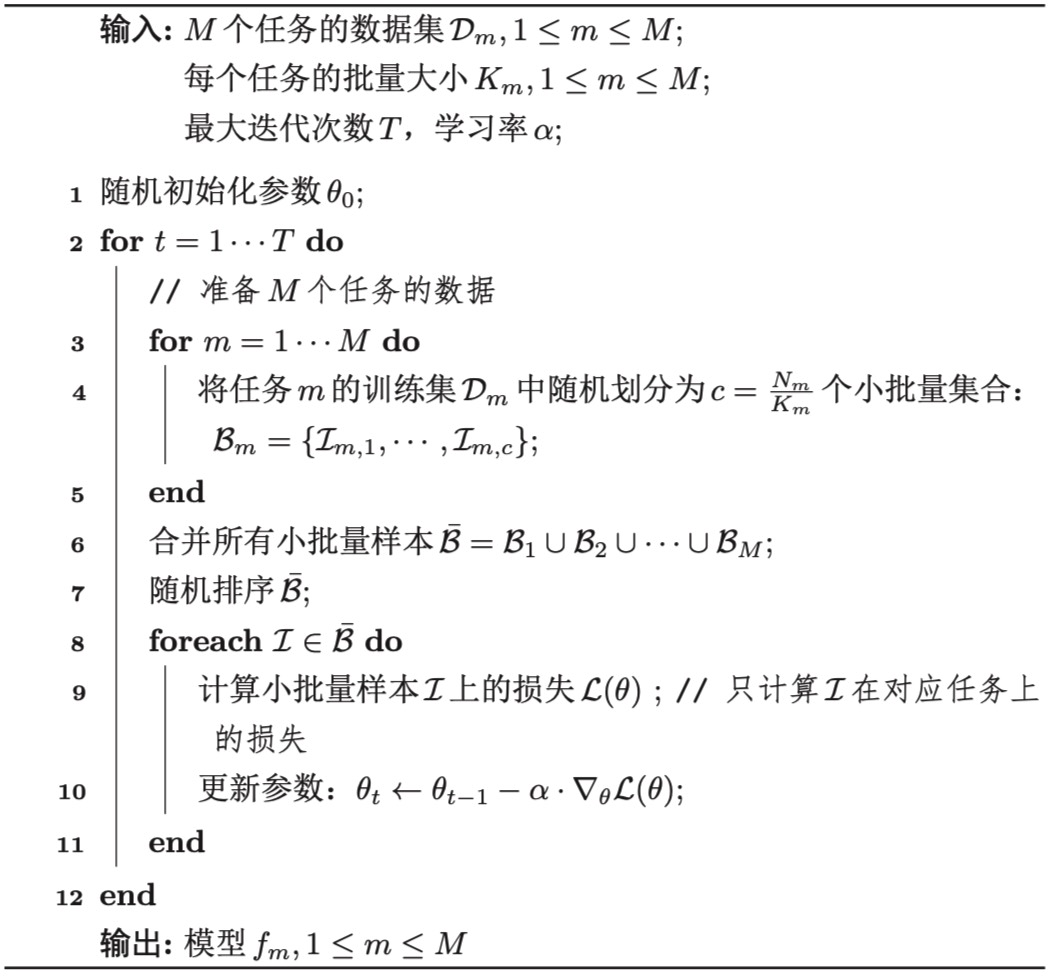• 1.多任务学习在多个数据集上进行训练，训练集范围更大，且多任务之间具有一定的相关性，相当于是一种隐式的数据增强，可以提高模型的泛化能力。
• 2.多任务学习中的共享模块需要兼顾所有任务，在一定程度上避免了模型过拟合到单个任务的训练集，可以看做是一种正则化。
• 3.多任务学习比单任务学习可以获得更好的表示
• 4.在多任务学习中，每个任务都可以“选择性”利用其他任务中学习到的隐藏特征，从而提高自身的能力。

### 迁移学习

#### 归纳迁移学习

• 当源领域只有大量无标注数据时，源任务可以转换为无监督学习任务，比如自编码和密度估计，通过无监督任务学习一种可迁移的表示，然后将这些表示迁移到目标任务上。

• 当源领域有大量的标注数据时，可以直接将源领域上训练的模型迁移到目标领域上。
归纳迁移学习一般有下面两种迁移方式：

• 基于特征的方式：将预训练模型的输出或者中间隐藏层的输出作为特征直接加入到目标任务学习模型中。目标任务的学习模型可以时一般的浅层分类器（比如支持向量机等）或一个新的神经网络模型。

• 精调的方式：在目标任务上复用预训练模型的部分参数，并对其参数进行精调。

• （1）初始模型的性能一般比随机初始化的模型要好
• （2）训练时模型的学习速度比从零开始学习要快，收敛性更好
• （3）模型的最终性能更好，具有更好的泛化性

• （1）多任务学习是同时学习多个不同任务，而归纳迁移学习通常分为两个阶段，即源任务上的学习阶段，和目标任务上的迁移学习阶段
• （2）归纳迁移学习是单向的知识迁移，希望提高模型在目标任务上的性能，而多任务学习时希望提高所有任务的性能。

#### 转导迁移学习

• （1）协变量偏移（Covariate Shift）：源领域和目标领域的输入边际分布不同$p_S(x) \neq p_T(x)$，但后验分布相同$p_S(y|x) = p_T(y|x)$，即学习任务相同$T_s = T_T$
• （2）概念偏移（Concept Shift）：输入边际分布相同$p_S(x) = p_T(x)$，但后验分布不同$p_S(y|x) \ neq p_T(y|x)$，即学习任务不同$T_S \neq T_T$
• （3）先验偏移（Prior Shift）：源领域和目标领域中的输出$y$先验分布不同$p_S(y) \neq p_T(y)$，条件分布相同$p_S(x|y) = p_T(x|y)$。在这样的情况下，目标领域必须提供一定数量的标注样本。

### 元学习

#### 基于优化器的元学习

$\theta_t \leftarrow \theta_{t-1} - \alpha \bigtriangledown L(\theta_{t-1})$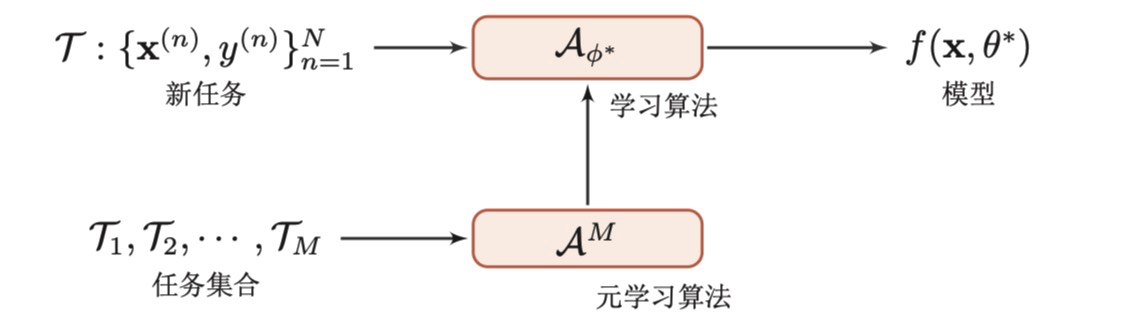$\theta_{t+1} = \theta_t + g_t(\bigtriangledown L(\theta_{t}), \phi )$

$L(\phi) = E_f [ \sum_{t=1}^{T} w_t L(\theta_t) ] \\ \theta_t = \theta_{t-1} + g_t \\ [g_t: h_t] = LSTM( \bigtriangledown L(\theta_{t-1}), h_{t-1}, \phi)$

#### 模型无关的元学习

$\theta_m ' = \theta- \alpha \bigtriangledown _\theta L_{T_m}(f_\theta)$

MAML的目标是学习一个参数$\theta$使得其经过一个梯度迭代就可以在新任务上达到最好的性能。
$\underset{ \theta }{ min } \sum_{T_m \sim p(T)} L_{T_m}(f(\theta'_m)) = \sum_{T_m \sim p(T)} L_{T_m} ( f(\theta - \alpha \bigtriangledown _\theta L_{T_m} (f_\theta )) )$

$\theta \leftarrow \theta - \beta \bigtriangledown _\theta \sum_{m=1}^{M} L_{T_m}(f_{\theta_m'})$

MAML的具体过程算法如下：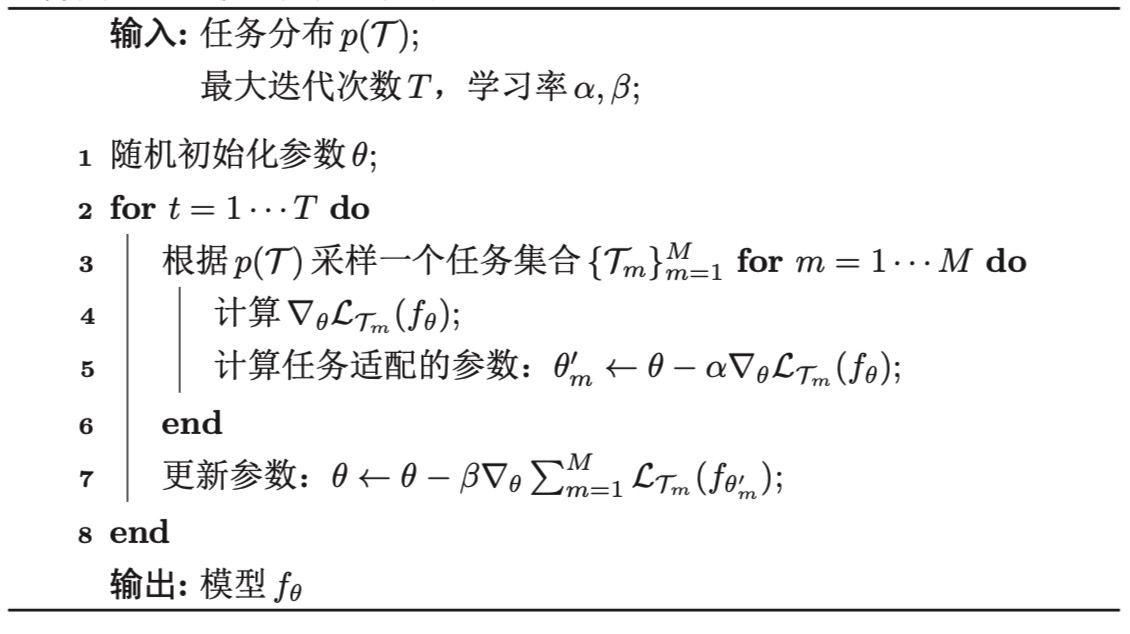### 总结

【技术服务】，详情点击查看： https://mp.weixin.qq.com/s/PtX9ukKRBmazAWARprGIAg#### 一文看懂深度学习（白话解释+8个优缺点+4个典型算法）

07-1306-131208

#### 概率图模型8：独立性

05-011050

#### 如何优化深度学习模型

08-25161

#### 关于模型的独立性

02-031135

#### 手把手教你如何部署深度学习模型

06-292411

#### 游戏建模师经验分享：模型学习方法

05-30353

#### 模型独立学习：多任务学习与迁移学习

03-1524

09-207003

#### 常用的深度学习模型

06-26195

#### 模型的学习方式-迁移学习、多任务学习、小样本学习、元学习

02-011737

#### 时空序列预测模型之PredRNN（用ST-LSTM的预测学习循环神经网络）

01-122万+

#### 多任务学习

04-16248

#### 广告CTR预估中用户行为学习和记忆建模

03-1558

#### RS Meet DL(80)-在评分预测中建模“同化-对比”效应

05-23314

#### 推荐系统遇上深度学习(八十三)-[阿里]记忆增强网络—MA-DNN模型介绍

05-23261

03-14235

#### 第十章 模型独立的学习方式

03-161276

#### Qt学习之路---模型与自定义模型

02-1928万+

#### 软件测试入门、SQL、性能测试、测试管理工具

03-02©️2020 CSDN 皮肤主题: 大白 设计师: CSDN官方博客点击重新获取扫码支付1.余额是钱包充值的虚拟货币，按照1:1的比例进行支付金额的抵扣。
2.余额无法直接购买下载，可以购买VIP、C币套餐、付费专栏及课程。余额充值